﻿ Area of a Triangle

# AREA OF A TRIANGLE

• PRACTICE (online exercises and printable worksheets)

An earlier section, Area Formulas: Triangle, Parallelogram, Trapezoid , showed how to find the area of a triangle with a base/height pair. The key results are summarized here for convenience.

With trigonometry now in hand, another useful formula for the area of a triangle is given.

## AREA OF A TRIANGLE USING A BASE/HEIGHT PAIR

 Take a vertex of a triangle. Drop a perpendicular to the opposite side or (if needed) to an extension of the opposite side. The segment just created is called a height or altitude of the triangle. The side opposite the vertex is called the corresponding base. Since every triangle has three vertices, every triangle has three base/height pairs. Area is half base times height: $$\begin{gather} \text{area of triangle}\cr \text{with base \,b\, and height \,h\,}\cr = \frac 12 bh \end{gather}$$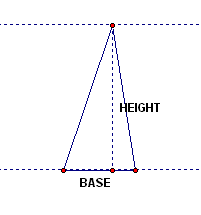Drop a perpendicular to the opposite side ...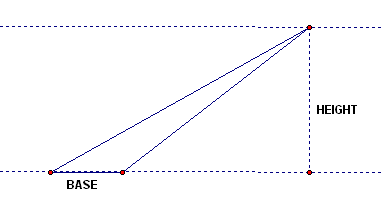... or, to an extension of the opposite side.

 A derivation of the triangle area formula ($\,bh/2\,$) was given in Area Formulas: Triangle, Parallelogram, Trapezoid for the situation where the altitude ‘hits’ the base (left-most picture above). The formula also works when the altitude doesn't ‘hit’ the base, but instead hits an extension of the base, as shown at right. Here's how: Suppose you want the area of the blue triangle at right ($\,\triangle ABC\,$). This triangle has base $\,b\,$ and height $\,h\,$. Let $\,x\,$ denote the length of the red-dashed segment. Then: \begin{align} &\text{area of blue triangle}\cr\cr &\qquad = \quad \text{area of big right triangle } (\triangle ABD) - \text{area of small right triangle } (\triangle CBD)\cr\cr &\qquad = \quad \frac 12(b+x)h - \frac 12 xh\cr\cr &\qquad = \quad \frac 12bh + \frac 12xh - \frac 12xh\cr\cr &\qquad = \quad \frac 12bh \end{align}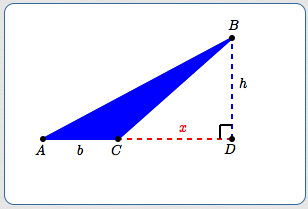area of blue triangle is $\,\frac 12 bh\,$

Note that there are infinitely many triangles with a given base/height pair.
For example, all the triangles below have the same base/height pair.
Same base/height pair—same area!
A base/height pair uniquely determines the area of a triangle, but not the shape of the triangle.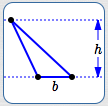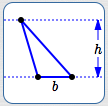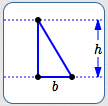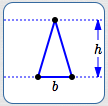All these triangles have the same base/height pair, hence the same area.

## AREA OF A TRIANGLE USING TWO SIDES AND AN INCLUDED ANGLE

By the SAS (side-angle-side) triangle congruence theorem,
two sides and an included angle uniquely defines a triangle.

Let $\,a\,$ and $\,b\,$ denote two sides of a triangle,
with included angle $\,\theta\,$.

The sketches at right and below show the two situations that can occur when finding the altitude that has corresponding base $\,b\,$. In both cases, the altitude length is found using the yellow triangle (which is a right triangle with hypotenuse $\,a\,$).

In both cases, the altitude length is $\,h = a\sin\theta\,$.

Thus: \begin{align} &\text{area of triangle with sides a and b and included angle \theta}\cr\cr &\qquad = \frac 12(\text{base})(\text{height})\cr\cr &\qquad = \frac 12 b(a\sin\theta)\cr\cr &\qquad = \frac 12 ab\sin\theta \end{align} In words, the area is:

half the product of the two sides,
times the sine of the included angle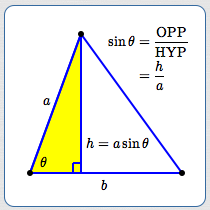(When we write $\,180^\circ - \theta\,$, it is assumed that $\,\theta\,$ is measured in degrees.)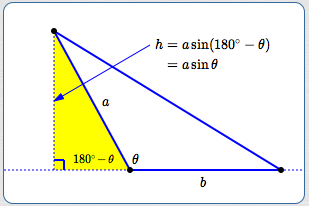Master the ideas from this section